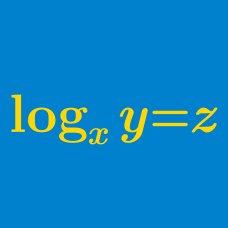Algebra

# Logarithmic Functions Problem Solving

Let $$w, x, y, z$$ be positive reals greater than 1, and

$\log_x w = 24, \log_y w = 40, \log_{xyz} w = 12 .$

Evaluate $$\log_z w$$.

The only real solution of the equation $\ln x + \ln (x^2-34)=\ln 72$ can be uniquely written in the form $$a+\sqrt{b}$$ for some integers $$a$$ and $$b$$. Find $$a+b$$.

Given that $$\log_{10}2=0.3010$$, $$\log_{10}3=0.4771$$ and $$\log_{10}7=0.8451$$, let $$a$$ be the number of digits in $$3^{62}$$. If $$b$$ is the first digit fromthe left of $$3^{62}$$, what is $$a+b$$?

Let $$N$$ be the number of times that the first digit of $$2^n$$ is $$1,$$ with $$n$$ being an integer such that $$0\leq n \leq 10^{10}$$. What are the last three digits of $$N?$$

You may use the fact that
$0.3010299956639811952 < \log_{10} 2 < 0.3010299956639811953$

Evaluate $\lfloor \log_{8} 1 \rfloor + \lfloor \log_{8} 2 \rfloor + \lfloor \log_{8} 3 \rfloor + \cdots + \lfloor \log_{8} 154 \rfloor.$

Details and assumptions

Greatest Integer Function / Floor Function: The function $$\lfloor x \rfloor: \mathbb{R} \rightarrow \mathbb{Z}$$ refers to the greatest integer smaller than or equal to $$x$$. For example, $$\lfloor 2.3 \rfloor = 2$$ and $$\lfloor -5 \rfloor = -5$$.

×